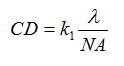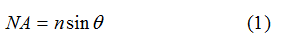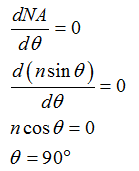# What is the smallest possible feature size that can be resolved by using a 193-nm optical source and the highest possible NA that can be obtained by a lens when exposing the image in air? Note that NA can be determined by the equationNA = n∙sin(θ)where n is the index of refraction of the medium in which the lens is working (air in this instance) and θ is the maximal half-angle of the cone of light that can enter or exit the lens and has a maximum possible value of 90˚ for a very large lens.

Question
1 views

What is the smallest possible feature size that can be resolved by using a 193-nm optical source and the highest possible NA that can be obtained by a lens when exposing the image in air? Note that NA can be determined by the equation

NA = n∙sin(θ)

where n is the index of refraction of the medium in which the lens is working (air in this instance) and θ is the maximal half-angle of the cone of light that can enter or exit the lens and has a maximum possible value of 90˚ for a very large lens.

check_circle

Step 1

The smallest possible feature size, that is the critical dimension (CD) can calculate by the following expression.Step 2

Write the expression for the numerical aperture (NA) of the lens.Step 3

Calculate the angle (θ) for the maximum numerical aperture (NAmax) as below,...

### Want to see the full answer?

See Solution

#### Want to see this answer and more?

Solutions are written by subject experts who are available 24/7. Questions are typically answered within 1 hour.*

See Solution
*Response times may vary by subject and question.
Tagged in